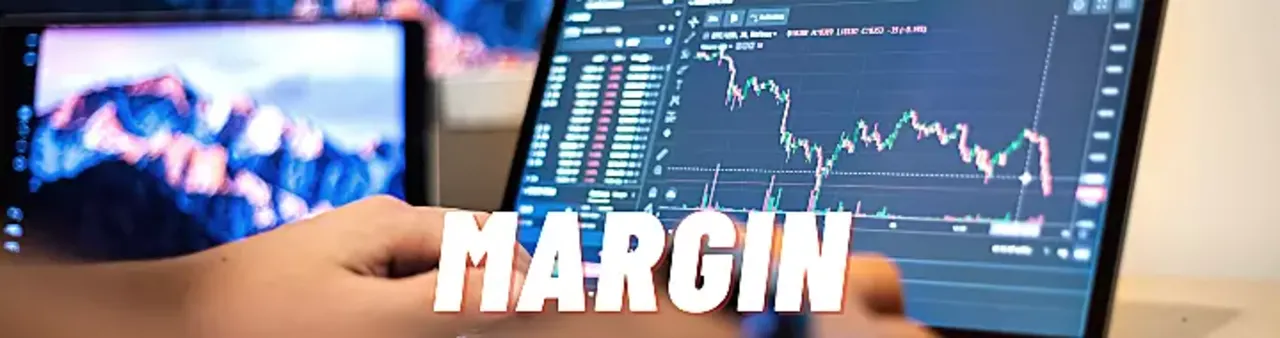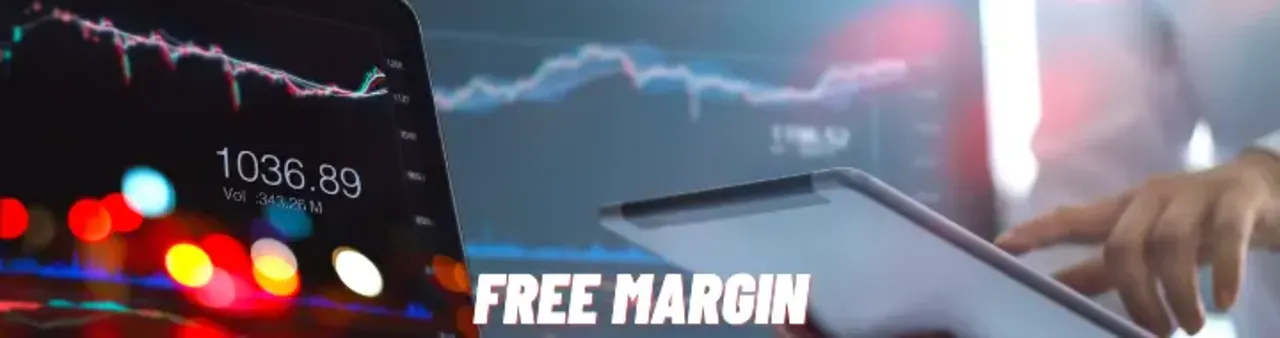# What is Margin in Forex Trading?

Daksh Murkute | | |

Understanding margin is very important for traders as a lot of different things depend upon it. If a person does business and doesn't know a thing about the basic accounts, sooner or later it is going to cost him a lot of money.

In the same way, forex trading is also a business and it is very important to understand the simple accounts of forex trading, which is margin, leverage, and a few other essential things.

In this blog post, I will talk about what is margin and how is it important.

### Contents

What is margin?

Margin requirement

Required margin

How to calculate required margin?

What is free margin?

What is margin level and margin calls?

#### Key Takeaways

###### iii. Margin call is when the loss in the trade has to exceed the margin and the trader needs to deposit more margin.

Margin is the amount that traders have to deposit with their broker for the trades they take. This margin amount is usually just a fraction of the total value of the trade and it's there because of the high leverages that forex brokers give to traders.

## What is margin?Simply, the margin is a good faith deposit that a trader gives to a broker to initiate a trade.

This amount that the broker sets aside or locks is called the margin.

The calculation of margin depends upon various things like the base currency of your account, the currency pair you are trading, leverage, and many other things.

But the margin is directly linked with the leverage. Let's try to understand this with the help of an example.

Example 1: Let us consider, you have a \$1000 account with a leverage of 1:100. This means that with \$1000 you can control a \$1,00,000 account, or you can buy/sell 1,00,000 units.

Now, if you decide to buy or sell 1,00,000 units of any currency, it means you are using 100% of your account and so, your broker will set aside \$1000 (which is 100% of your account). This simply means that your margin will be \$1000.

In the same way, if you decide to buy/sell only 10,000 units of any currency, it means you are using 10% of your account and so, your broker will set aside \$100 (10%of \$1000) from your account. So, now the margin is \$100.

Example 2: Let's say, you have the same \$1000 account but this time with the leverage of 1:50 i.e with an account of \$1000 you can now control \$50,000, which is 50 times more. Now, you decide to buy/sell 10,000 units of any currency,

It means you are using 20% of your account and hence the broker will keep that amount aside as a guarantee, 20% of \$1000 is \$200. So, your margin will be \$200.

In the same way, if you buy or sell 50,000 units with this account the margin will be \$1000.

You can now easily understand what margin is and how it is related to leverage. But, there are various other things related to margin which is important for a forex trader.

## Margin requirement

The amount of margin required to open a position varies from broker to broker and currency pair to currency pair and also the leverage your broker is providing you.

Depending upon the broker you use there are different margin requirements for each broker such as 0.25%, 0.5%, 1%, 2% or more. This percentage is called the margin requirement.

So, in simple terms margin requirement is the amount of money your broker requires from you to open a position.

## Required margin

When margin is expressed as a specific amount of your account’s currency, this amount is known as the required margin.

Or in simple terms, the margin amount required to open a position is called as required margin.

Every position you open with your account will have its required margin which will be locked up by the broker.## How to calculate the required margin?

Calculating the required margin depends upon the currency pair you are trading, your base currency, the leverage you are using, and the exchange rate if the base currency is different than the actual account currency.

To help you understand this easily let's breakdown a few basic things.

Base currency: In any currency pair, the currency which comes first is called the base currency. For example, in EURUSD, EUR is the base currency.

Account currency: It is the primary currency in which your account is made.

Now, let's move forward and understand how to calculate the required margin.

There are two different formulas for calculating the required margin.

### 1. When base currency is the same as the accounts currency

Required margin = Total no. of units X Exchange rate/100

Note - When base currency is the same as the account currency the exchange rate will be 1.

For example, let's say, your account currency is USD. And you deposited your account at \$1000 with a leverage of 1:100. Now, you want to go long/ buy USDJPY with a lot size of 0.10 (10,000 units)

Required margin = Total no. of units X Exchange rate / 100

= 10,000 X 1 / 100

= \$100

So, the required margin for opening a trade with 0.10 lot will be \$100.

### 2. When base currency is different from the account's currency

Required margin = Total no. of units X Exchange rate/100

Note - When base currency is different from the account currency the exchange rate will depend upon the current exchange rate provided by the broker.

Example 1: Let's say, your account currency is USD and you deposited your account at \$1000 with a leverage of 1:100. Now, you want to go long/ buy GBP-USD with a lot size of 0.10 (10,000 units), which means you want to buy pounds.

Required margin = Total no. of units X Exchange rate / 100

= 10,000 X 1.20161 / 100

= \$120.16

Note - Here exchange rate provided by the broker to buy pound was 1.20161.

Example 2: Let's say, your account currency is USD. And you deposited your account at \$1000 with a leverage of 1:100. Now, you want to go long/ buy AUD-CAD with a lot size of 0.10 (10,000 units), which means you want to buy Australian dollars.

So, here the exchange rate provided by the broker is 0.59390.

Required margin = Total no. of units X Exchange rate / 100

= 10,000 X 0.59390 / 100

= \$59.39

The only reason for having funds in your account is to make sure you have enough margin to use for trading.

When it comes to trading forex, your ability to open trades is not necessarily based on the funds in your account balance but the amount of margin you have left with.

Was this method of calculating the required margin was too confusing for you or are you worried about the formulas involved? Don't worry. Almost every broker provides a tool by which you can easily calculate the required margin.

Below I have provided you with a link that will help you calculate the required margin in no time.## What is free margin?

The free margin in forex is the amount of money that is not involved in any trade or the total unused margin left with you is called the free margin.

You can use this free margin to take more positions. Technically, the free margin is the difference between equity and margin.

Free margin = Equity - Margin

The free margin directly depends upon your equity, that is if you have few open positions and if those positions are in profit, the more profit they make, the greater the equity you will have.

As the equity will rise, the free margin will also rise. This means that the free margin increases if the equity increases and decreases if the equity decreases.

Now, you may be wondering, what happens if you don't have enough free margin? If you don't have a free margin, you won't be able to place new trades.

Now that you have understood free margin, let's see what is a margin level?

## What is margin level and margin calls?

The margin level is the percentage value based on the amount of accessible free margin (equity) versus used margin. In other words, it is the ratio of equity to used margin.

Margin level = (equity/used margin) x 100.

Brokers use margin levels to identify whether FX traders can take any new positions or not.

Different brokers have differing limits for the margin level, but most of them set this limit at 100%. This limit is called a margin call level.

Now, different forex broker have different margin call levels, some keep it to 100% and some keep it to 50%.

As your accounts margin level moves to 100% you are not allowed to take any new trades, though you are allowed to cancel the previous trades.

As long as your equity level remains above the free margin, you will not receive a margin call. 100% margin call levels occur when your account equity is equal to the margin.

This happens when you have losing positions and the market is going against you. So, when your account's equity equals the account's margin, you are not able to place any new trade.

Now let's say, your account has already hit the 100% margin call level and went below it, now as the margin level goes below it, the broker will automatically start closing all your losses starting from your highest loss.

This limit at which your losses are automatically closed is called the stop out levels. It is done to make sure that you don't lose more than the amount you have deposited in your account.